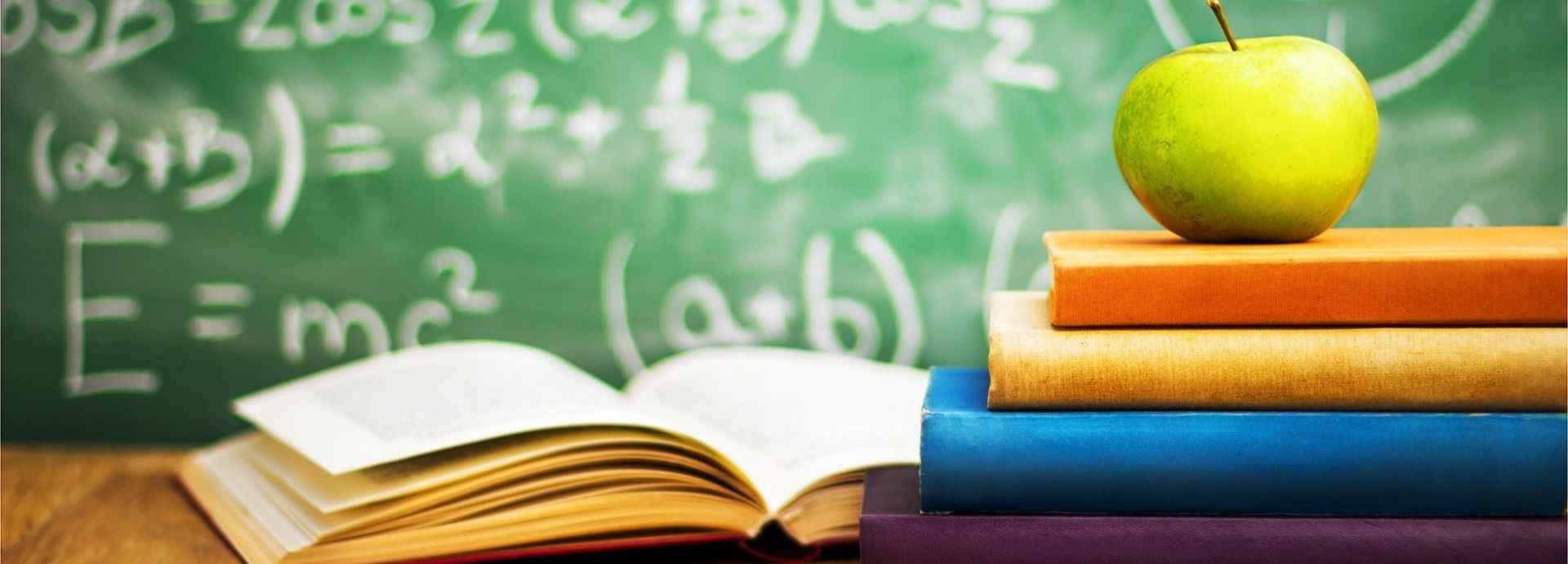# Math tutors in Toronto | Vancouver | CalgaryMath is the no doubt the toughest subject for the greater percentage of students. If your child is not good in math, then classroom teaching may not be the method by which he/she can learn. In such cases, your child needs an experienced and qualified math tutor for his math learning. It has also been proven that one-to-one method is the best way of learning especially when it comes to mathematics. Personal attention makes all the difference
and enables your child to know his way of learning. Thus, hiring a math tutor for your child will not only enhance his skills in mathematics but it will also make the subject interesting for your child when the tutor teaches him/her in creative ways.

## Math tutoring in Calgary Alberta cirriculum

In Alberta, they are separated into -1 and -2 Course Sequences:

Math 10C • involves topics such as measurement, powers, irrational numbers, functions and relations, and trigonometry

• focuses on algebraic skills and reasoning

• provides several choices
after you successfully complete it and move to 20-level courses

Math 20-1, 30-1

• investigate the relationships between relations and functions, and engage in trigonometric topics

• develop abstract reasoning and visualization in a problem-solving environment
• prepare you for a post-secondary program that requires calculus

Math 20-2, 30-2

• focus on investigation into logical reasoning, relations and functions, and trigonometry

• develop problem-solving, reasoning, and various ways of communicating mathematical
understandings, including a research project

• prepare for a post-secondary program that does not require calculus

## Math tutoring in Vancouver British Columbia curriculum

In the Bristish Columbia math curriculum, most of the students will use either the Foundations of
Mathematics or Pre-calculus pathway.

Foundations of Mathematics: This pathway is designed to provide students with the mathematical understandings and critical-thinking skills identified for post-secondary studies in programs that do not
require the study of theoretical calculus. Topics include financial mathematics, geometry, measurement, number, logical reasoning, relations and functions, statistics and probability.

Pre-calculus: This pathway is designed to provide students with the mathematical understandings and critical-thinking skills identified for entry into post-secondary programs that require the study of theoretical calculus. Topics include algebra and number, measurement, relations and functions, trigonometry, and permutations, combinations and binomial theorem.